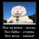6191 obejrzeń
Continue to experiement with VWAP and EVWMA.
It seems that just simple crosses between VWAP and EVWMA can be pretty good signals. VWAP is a bit choppy so you can use VWAP smoothing input to smoth it a bit.

Here are few other strategies based on EVWMA:

EVWMA VWAP MACD Strategy

Courses:
PineScript Programming: https://qntly.com/pinepro

Discord: https://qntly.com/discord
Telegram: https://qntly.com/tel

## KomentarzeI added a time period for the test, everything is not so rosy, sorry if you set ... test since 2019 year..

//@version=4
strategy("EVWMA VWAP Cross ", shorttitle="EVWMA_VWAP_Cross", overlay=true)

// Inputs
sum_length = input(30, title = "EVWMA Length", type = input.integer)
vwap_smoothing = input(1, title = "VWAP Smoothing", type = input.integer)
//set time
year_start= input(2017, minval=1)
month_start= input(1, minval=1)
day_start= input(1, minval=1)

year_finish= input(2100, minval=1)
month_finish= input(1, minval=1)
day_finish= input(1, minval=1)

tt0=timestamp(year_start,month_start,day_start,23,59)
tt1=timestamp(year_finish,month_finish,month_finish,23,59)

tim=time

metka= tim>tt0 and tim<tt1 ? true : false
//other

// Calculate EVWMA
vol_period = sum(volume, sum_length)

evwma = 0.0
evwma := ((vol_period - volume) * nz(evwma, close) + volume * close) / (vol_period)

vwap_smooth = ema(vwap, vwap_smoothing)

long = crossover( vwap_smooth, evwma)
short = crossunder(vwap_smooth, evwma)

plot(evwma, color = color.blue)
plot(vwap_smooth, color = color.red)

strategy.entry("Long", true, when = long and metka )
strategy.entry("Short", false, when = short )
Odpowiedz